# NCERT Solutions for Class 8 Maths Chapter 11 Mensuration Ex 11.4

NCERT Solutions for Class 8 Maths Chapter 11 Mensuration Exercise 11.4

Ex 11.4 Class 8 Maths Question 1.
Given a cylindrical tank, in which situation will you find the surface area and in which situation volume.(a) To find how much it can hold.
(b) Number of cement bags required to plaster it.
(c) To find the number of smaller tanks that can be filled with water from it.
Solution:
(a) In this situation, we can find the volume.
(b) In this situation, we can find the surface area.
(c) In this situation, we can find the volume.

Ex 11.4 Class 8 Maths Question 2.
Diameter of cylinder A is 7 cm, and the height is 14 cm. Diameter of cylinder B is 14 cm and height is 7 cm. Without doing any calculations can you suggest whose volume is greater? Verify it by finding the volume of both the cylinders. Check whether the cylinder with greater volume also has greater surface area?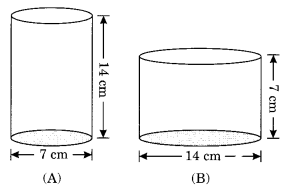Solution:
Cylinder B has a greater volume.
Verification:
Volume of cylinder A = πr2hEx 11.4 Class 8 Maths Question 3.
Find the height of a cuboid whose base area is 180 cm2 and volume is 900 cm3.
Solution:
Given: Area of base = lb = 180 cm2
V = 900 cm3
Volume of the cuboid = l × b × h
900 = 180 × h
h = 5 cm
Hence, the required height = 5 cm.

Ex 11.4 Class 8 Maths Question 4.
A cuboid is of dimensions 60 cm × 54 cm × 30 cm. How many small cubes with side 6 cm can be placed in the given cuboid?
Solution:
Volume of the cuboid = l × b × h = 60 cm × 54 cm × 30 cm = 97200 cm3
Volume of the cube = (Side)3 = (6)3 = 216 cm3
Number of the cubes from the cuboid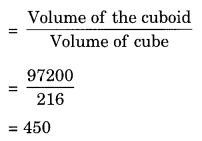Hence, the required number of cubes = 450.

Ex 11.4 Class 8 Maths Question 5.
Find the height of the cylinder whose volume is 1.54 m3 and the diameter of the base is 140 cm.
Solution:
V = 1.54 m3, d = 140 cm = 1.40 m
Volume of the cylinder = πr2h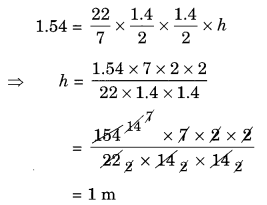Hence, the height of cylinder = 1 m.

Ex 11.4 Class 8 Maths Question 6.
A milk tank is in the form of a cylinder whose radius is 1.5 m and length is 7 m. Find the quantity of milk in litres that can be stored in the tank.Solution:
Here, r = 1.5 m
h = 7 m
.’. Volume of the milk tank = πr2h
= $$\frac { 22 }{ 7 }$$ × 1.5 × 1.5 × 7
= 22 × 2.25
= 49.50 m3
Volume of milk in litres = 49.50 × 1000 L (∵ 1 m3 = 1000 litres)
= 49500 L
Hence, the required volume = 49500 L.

Ex 11.4 Class 8 Maths Question 7.
If each edge of a cube is doubled,
(i) how many times will it be surface area increase?
(ii) how many times will its volume increase?
Solution:
Let the edge of the cube = x cm
If the edge is doubled, then the new edge = 2x cm
(i) Original surface area = 6x2 cm2
New surface area = 6(2x)2 = 6 × 4x2 = 24x2
Ratio = 6x2 : 24x2 = 1 : 4
Hence, the new surface area will be four times the original surface area.

(ii) Original volume of the cube = x3 cm3
New volume of the cube = (2x)3 = 8x3 cm3
Ratio = x3 : 8x3 = 1 : 8
Hence, the new volume will be eight times the original volume.

Ex 11.4 Class 8 Maths Question 8.
Water is pouring into a cuboidal reservoir at the rate of 60 litres per minute. If the volume of the reservoir is 108 m3, find the number of hours it will take to fill the reservoir.
Solution:
Volume of the reservoir = 108 m3 = 108000 L [∵1 m3 = 1000 L]
Volume of water flowing into the reservoir in 1 minute = 60 L
Time taken to fill the reservoir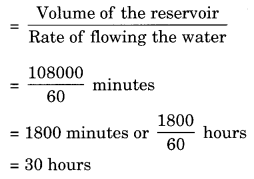Hence, the required hour to fill the reservoir = 30 hours.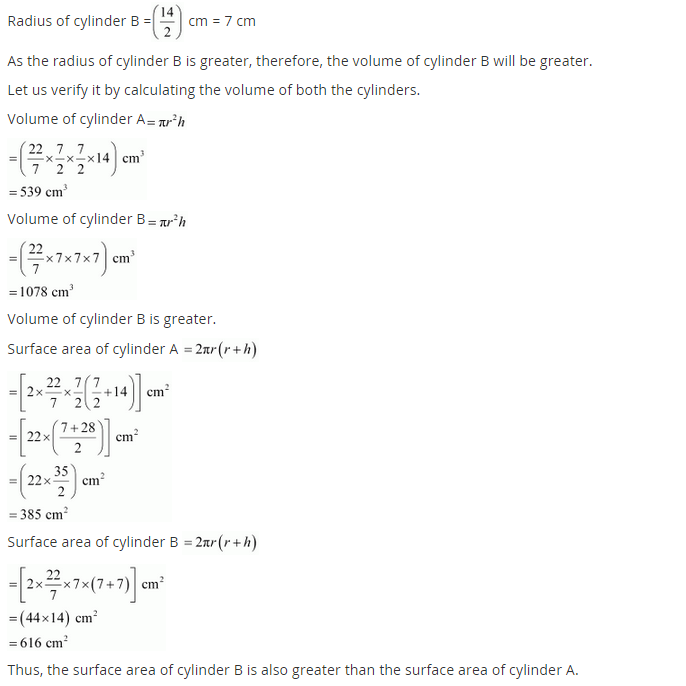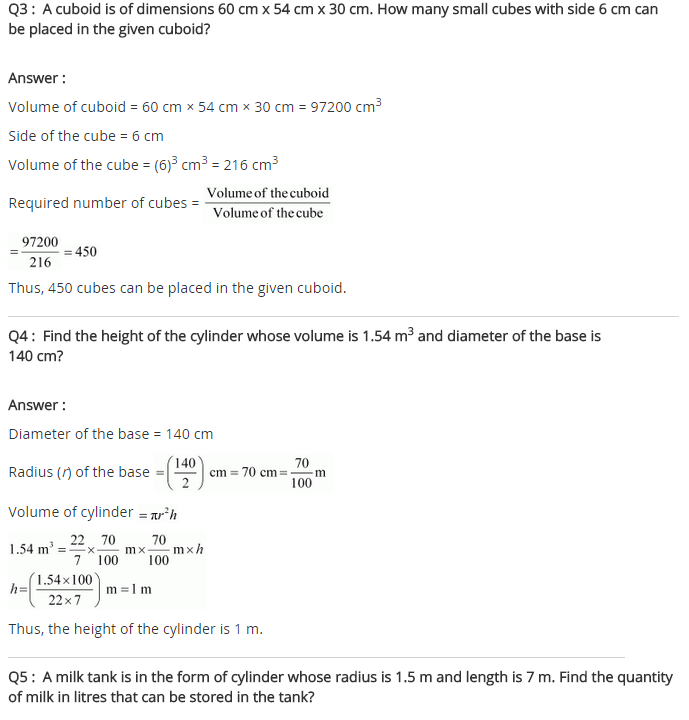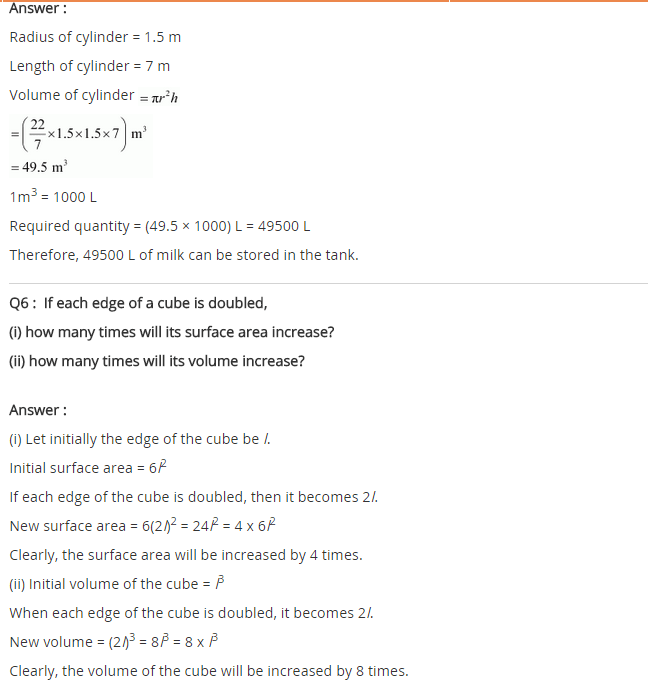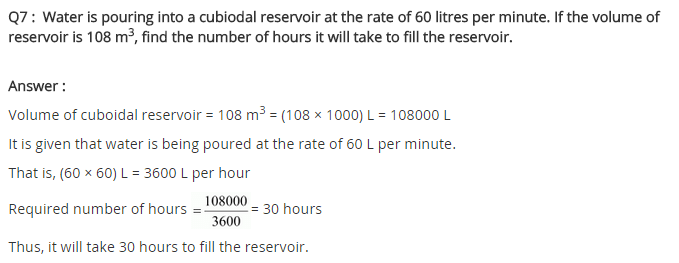+